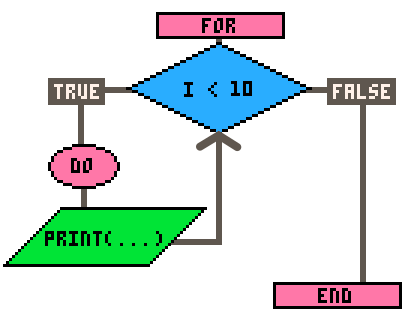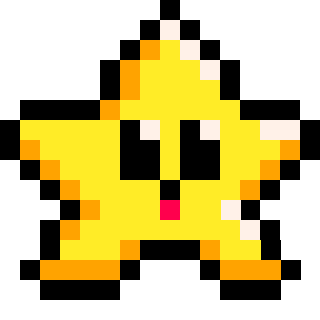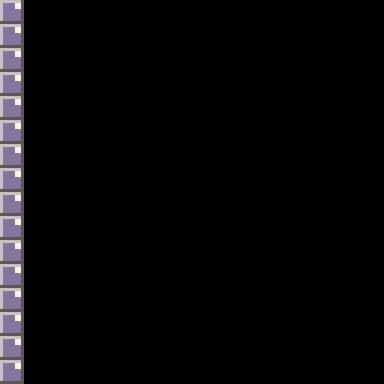# LOOP

A loop is a feature of programming that allows you to repeat a specific block of code multiple times until receiving the order to stop. They are useful when you need to perform a task multiple times, or when you need to iterate (go over) a set of data. Most loops will repeat until a certain condition is met.

The most common loop is the `for` loop.

``````for i=0, 10, 1 do
print(i)
end

--prints 0 1 2 3 4 5 6 7 8 9 10``````

Here is a flowchart to visualize this code:This loop keeps track of a variable (most use the letter `i` like this), which we set to start at `0`, and at the end of each repitition it increases by the third number, `1`. Before starting the next repitition, it checks the condition and compares `i` with the second number given, `10`. If `i` is less than `10`, then the condition is true and the loop continues. Only when the condition is false, does the loop end.

There are different types of loops that are unique in how they handle what they repeat and when they stop, so it is important to learn them so you can choose the best type of loop for what you need.#### Types of Loops

For - used to execute a block of code a fixed number of times, typically by iterating over a range of values.

While - used to execute a block of code repeatedly as long as a certain condition is true.

Foreach - used to iterate over the entries of a table and pass each entry to a function.

For in all - used to iterate over all entries of a numeric table and set each entry as a local variable.

For in pairs - used to iterate over the key-value pairs of a table.

Repeat Until - similar to a while loop, but it executes the block of code at least once, and then repeats it until a certain condition is true.#### Common Uses of Loops

Loops are often used with sets of data (tables) to update, compare, or draw each entry in the set. Loops are helpful to simplify complex code. This is useful in:

Physics - To apply forces such as gravity and friction to multiple objects' movement variables, you can use a loop.

``foreach( object, apply_gravity )``

Animation - To cycle through sprites for multiple objects, you can use a loop.

``for f in all(flowers) do f.sprite+=1 end``

Particle systems - To change the position, size, or color of each particle in a table, you can use a loop.

``foreach( particle, update_particles )``

Pathfinding - To compare multiple enemy with tiles on the map to determine the fastest route, you can use a loop inside another loop.

``````for e in all(enemies) do
for t in all(tiles) do
--compare each enemy data with each tile data
end
end``````

Collision Detection - To check collision of a player with a list of bullets, you can use a loop.

``````for key,bullet in pairs(bullets) do
if player.x==bullet.x and player.y==bullet.y then
--player hit
end
end``````

Procedural Generation - To build random levels and maps using only code, you can use a couple loops.

``````for column=1, 16 do
for row=1, 16 do
--draw certain background tile
end
end``````#### Code Efficiency with Loops

Whenever you are copy/pasting code, consider how you could use a loop instead.

For example, we could draw wall tiles on the left side of the screen like this:``````local x=0
local y=0
local tile_size=8

spr(1, x, y + tile_size * 0)
spr(1, x, y + tile_size * 1)
spr(1, x, y + tile_size * 2)
spr(1, x, y + tile_size * 3)
spr(1, x, y + tile_size * 4)
spr(1, x, y + tile_size * 5)
spr(1, x, y + tile_size * 6)
spr(1, x, y + tile_size * 7)
spr(1, x, y + tile_size * 8)
spr(1, x, y + tile_size * 9)
spr(1, x, y + tile_size * 10)
spr(1, x, y + tile_size * 11)
spr(1, x, y + tile_size * 12)
spr(1, x, y + tile_size * 13)
spr(1, x, y + tile_size * 14)
spr(1, x, y + tile_size * 15)

--20 lines, 153 tokens``````

Or, we could simply use a loop to do the exact same thing, like this:``````local tile_size = 8
local x = 0
local screen_height = 128

for row = 0, screen_height/tile_size-1 do
local y = row * tile_size
spr(1, x, y)
end

--8 lines, 31 tokens``````

Notice how the longer code was copy pasted many times, and the only thing that changed in each line was the number multiplied at the end. The for loop counts up automatically, just like the number we want to multiply. So we can use a for loop and set the interation number to a variable named `row` and simply multiply each row by the tile size to make the y position one more tile lower each time the loop repeats.

Not only are the number of lines 60% less, but the number of tokens used in PICO-8 is 80% less. In almost all cases, using loops will greatly reduce the amount of code you write and tokens you use.

If you are just starting out and learning to use loops. Start by writing out the long code by copy and pasting many lines yourself to get it working the way you want it. Then practice trying to convert that code into a loop. That is a great way to learn because eventually, you will be able to foresee when you should use a loop, and figure out how to use it before writing it all out first.

Writing inefficient code, then making it more efficient is a never-ending practice among programmers, so don't get stuck worrying if your first draft is the most efficient it can be. Just get it working first, then make it more efficient later.#### Beware of Infinite Loops

If not handled properly, a loop can cause the computer or a program to become slower or even crash as it becomes overwhelmed with repeating the same steps in an endless loop. Some causes for infinite loop crashes are:
(1) the condition to stop the loop is never met;
(2) your loop may not have any break points;
(3) the process is too long and complicated;
(4) the data set being looped through is too large.321

19 Apr 2023

`Font`